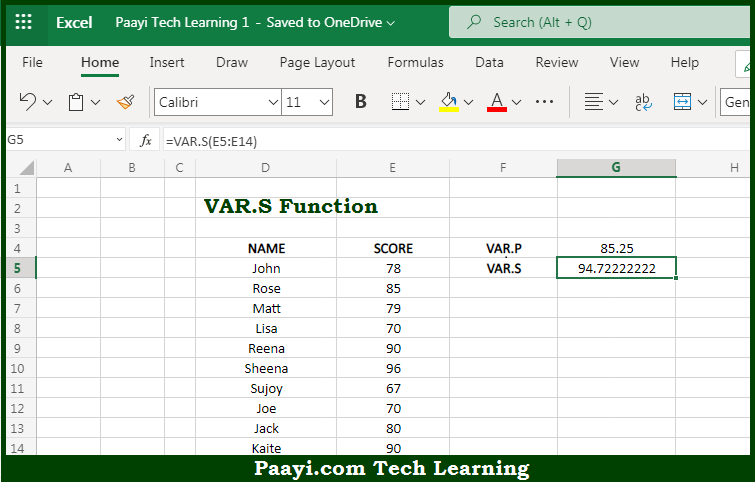# Learn How to Use Microsoft Excel VAR.S Function

Written by | 0 Comments | 658 Views

In this article, you will learn how to use the Microsoft Excel VAR.S function and its prime function in Microsoft Excel. You will also get to know the Microsoft Excel VAR.S function return value and syntax with the help of some examples.

Microsoft Excel VAR.S Function

The main purpose of the Microsoft Excel VAR.S function is to get the variation of the sample or population. That implies, with the help of the VAR.S function you can able to estimate the variance of the sample of a given data set or population. In case the data represents the entire population, you can use the VAR.P function. It should be noted that the VAR.S function ignores text values and the logical in the references. So, with the help of the VAR.S function, you can able to get the variation of the sample or population.

Return Value of VAR.S Function

The return value will be the calculated variance.

Syntax of VAR.S Function

=VAR.S(number1, [number2], ...)

Where the arguments:

• number1: This is the first number or reference.
• number2: This is the second number or reference (optional).

## How to Use Microsoft Excel VAR.S Function?So we know that Microsoft Excel VAR.S function you can able to get the variation of the sample or population. That implies, with the help of the VAR.S function you can able to estimate the variance of the sample of a given data set or population. In case the data represents the entire population, you can use the VAR.P function. It should be noted that the VAR.S function ignores text values and the logic in the references. So, with the help of the VAR.S function, you can able to get the variation of the sample or population.